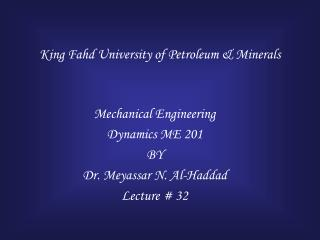# King Fahd University of Petroleum & Minerals - PowerPoint PPT PresentationDownload PresentationKing Fahd University of Petroleum & Minerals

King Fahd University of Petroleum & MineralsDownload Presentation## King Fahd University of Petroleum & Minerals

- - - - - - - - - - - - - - - - - - - - - - - - - - - E N D - - - - - - - - - - - - - - - - - - - - - - - - - - -
##### Presentation Transcript

1. King Fahd University of Petroleum & Minerals Mechanical Engineering Dynamics ME 201 BY Dr. Meyassar N. Al-Haddad Lecture # 32

2. General Application of the Equations of Motion Summation of moment in FBD = summation of the kinetic moment in K.D

3. Rectilinear Translation

4. Curvilinear Translation

5. x N slipping Vs. Tipping If x > 1.5 ft tipping If x < 1.5 ft slipping

6. Slipping Vs. Freely rotating mg FA=msNA NA NB

7. “Wheely” Vs. Non-Wheely Wheely : lift the front wheel off the ground NB=0

8. Example

9. Example mk=0.2 a= ? Flip Or Tipp? If x > 0.5 m tipping will take place

10. Example mm=125 kg mR= 75 kg a= ? NB=? FB=? ms=? Minimum to Lift front wheel

11. Example mBD=100kg mAB=mCD= Neglect q = 30o w = 6 rad/s TA= ? TD= ? aG=?

12. Problem aG mBNB NA NB m= 80 kg mB=0.8 NA=? NB=? When rear wheel locks for break a =? Deceleration

13. Problem a = 0 mBNB NA NB m= 80 kg mB= ? NA=? NB=? a =? When traveling at constant velocity and no break was applied

14. Problem a mkNA NA NB=0 m= 80 kg NA=? NB=? a =? mk=? minimum When rider applies the front break and back wheel start to lift off the ground

15. Thank You